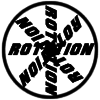#### You may also like### 8 Methods for Three by One

This problem in geometry has been solved in no less than EIGHT ways by a pair of students. How would you solve it? How many of their solutions can you follow? How are they the same or different? Which do you like best?### Rots and Refs

Follow hints using a little coordinate geometry, plane geometry and trig to see how matrices are used to work on transformations of the plane.### Reflect Again

Follow hints to investigate the matrix which gives a reflection of the plane in the line y=tanx. Show that the combination of two reflections in intersecting lines is a rotation.

# Matrix Meaning

##### Age 16 to 18 Challenge Level:
When thinking about rotations in three dimensions it may help to take an object and try turning it around different axes in different orders. What happens? How does this relate to what you've been asked to do?

This problem requires mathematical content found in the later Further Pure Mathematics A level modules.